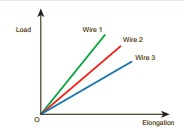# UNIT VII: Properties of Matter - Online Test

Q1. Consider two wires X and Y. The radius of wire X is 3 times the radius of Y. If they are stretched by the same load then the stress on Y is
Explaination / Solution:
No Explaination.

Q2. If a wire is stretched to double of its original length, then the strain in the wire is
Explaination / Solution:
No Explaination.

Q3. The load – elongation graph of three wires of the same material are shown in figure. Which of the following wire is the thickest?Explaination / Solution:
No Explaination.

Q4. For a given material, the rigidity modulus is (1/3) rd of Young’s modulus. Its Poisson’s ratio is
Explaination / Solution:
No Explaination.

Q5. A small sphere of radius 2cm falls from rest in a viscous liquid. Heat is produced due to viscous force. The rate of production of heat when the sphere attains its terminal velocity is proportional to
Explaination / Solution:
No Explaination.

Q6. Two wires are made of the same material and have the same volume. The area of cross sections of the first and the second wires are A and 2A respectively. If the length of the first wire is increased by ∆l on applying a force F, how much force is needed to stretch the second wire by the same amount?
Explaination / Solution:
No Explaination.

Q7. With an increase in temperature, the viscosity of liquid and gas, respectively will
Explaination / Solution:
No Explaination.

Q8. The Young’s modulus for a perfect rigid body is
Explaination / Solution:
No Explaination.

Q9. Which of the following is not a scalar?
Explaination / Solution:
No Explaination.

Q10. If the temperature of the wire is increased, then the Young’s modulus will
Explaination / Solution:
No Explaination.